Technological advancements have lead to reduced effort. This makes Python programming language as one of the most sought out programming language due to its ease of access and readability.  This also means a surge in the number of people experimenting with Python codes. In this blog, we will go through few of the most sought after fundamental python programs. Here is the list of same:

Palindrome Program In Python

A palindrome is a number, string or a sequence which will be the same even after we reverse the order. For example: MADAM, if spelled backwards will be same as MADAM.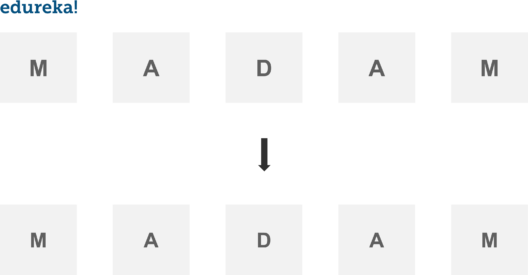Following is the python program to check whether the input string is a palindrome or not.
```
def pal(num):
x1 = num[::-1]
if x1 == x:
print('palindrome')
else:
print('not a palindrome')

print(pal('edureka'))
```

Factorial Program In Python

Factorial of a number is the product of all the positive numbers less than or equal to the number. The factorial of a number n is denoted by n!.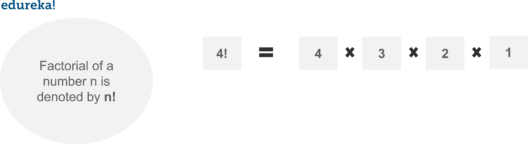Following is the program to find the factorial of a number.

```
def fact(num):
if num == 0:
return num
else:
return num * fact(num - 1)
print(fact(5))
```

Fibonacci Series Program In Python

A series in which the next term is the sum of the preceding two terms is called a fibonacci series. Fibonacci series is one of those python programs asked frequently in interviews.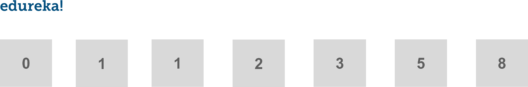Following is a program to print a fibonacci series.

```
a = int(input('enter the first element'))
b = int(input('enter the second element'))
n = int(input('enter the number of elements '))
print(a,b, end=" ")

while n-2:
c = a + b
a = b
b = c
print(c, end=" ")
n = n-1
```

Armstrong Number Program In Python

It is a special number, If we sum the cube of each of the digits in an armstrong number it will be equal to the number itself.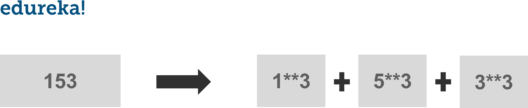Following is the python program to check whether a number is an armstrong number or not.

```
num = int(input('enter the nuber'))
s = 0
temp = num
while temp &gt; 0:
c = temp % 10
s += c**3
temp //= 10

if temp == num:
print('armstrong number')
else:
print('not an armstrong number')
```

Calculator Program In Python

When we think about a calculator, operations like addition, subtraction, multiplication and division comes into our mind. We will try to make a calculator program using python. We will add a few more operations in the program for better understanding.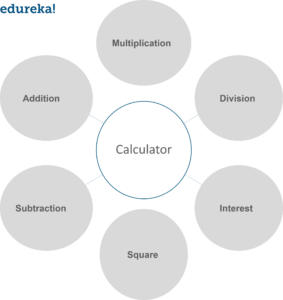Following is the program to create a calculator in python

```
return a+b

def sub(a,b):
return a-b

def prod(a,b):
return a * b

def div(a,b):
return a / b

def si(p, r, t):
return (p*r*t) / 100

def ci(p, r ,t):
return p * pow((1 + r/100), t)

def sqr(num):
return num**2

def sqrt(num)
return num**0.5

#to add two numbers, similarly you can use other functions for other operations.
```

Patterns Program In Python

We can use for loop to print various patterns in python. We will print a pyramid and a half pyramid pattern using the asterisk in a program.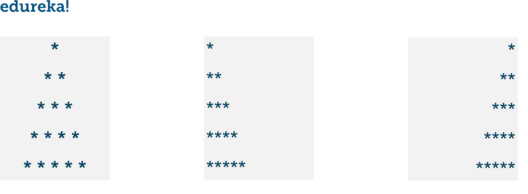Following is the program for patterns in python.

```
num = 5
a = (2 * num) - 2
for i in range(0, num):
for j in range(0, a ):
print(end=" ")
for j in range(0, i+1)
print('*' , end=" ")
print(" ")
```

Leap Year Program In Python

A leap year has 366 days with an extra day in the month of February. It occurs every four years. We will make a program to check if a year is a leap year or not?Following is the program to check if a year is a leap year

```year = int(input('enter year'))
if year % 400 == 0:
print('it is a leap year')
elif year % 4 == 0:
print('it is a leap year')
elif year % 100 == 0:
print('not a leap year')
else:
print('not a leap year')
```

Prime Number Program In Python

A prime number has only two factors, 1 and the number itself. We will make a program to check whether a number is a prime number or not.
Following is the program to check if the number is a prime number or not.
```num = int(input('enter number'))
for i range(2, num):
if  num% i == 0:
print('not a prime number")
break
else:
print('prime number')
break
```

Program To Print Areas In Python

We will take the following shapes and try to calculate the area of all these shapes in python.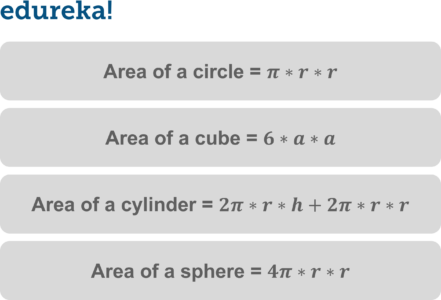Following is the program to calculate areas in python

```
import math
pi = math.pi

def cube(side):
return side**3

print(circle(10))
#You can use other functions to calculate the areas of other shapes.

```
Program To Reverse A List In Python
A list is a collection data type which is mutable and can contain duplicate elements as well. We will make a program to reverse a list in python.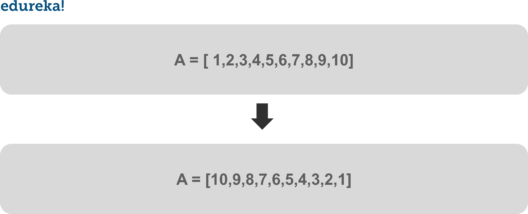Following is the program to reverse a list

```a = [1,2,3,4,5,6,7,8,9,10]
print(a[: : -1])
#this will print the list in reverse.
```
In this blog, we have programmed quite few logical topics in python. It is evident enough to prove how python differs from other programming languages and provides a much more efficient and easy to read coding platform.
This brings us to the end of this blog on python programs. To master your skills and python programming language and kickstart your learning, enroll to python certification program and discover the endless possibilities and applications of python programming language.

have any questions? mention them in the comments, we will get back to you.